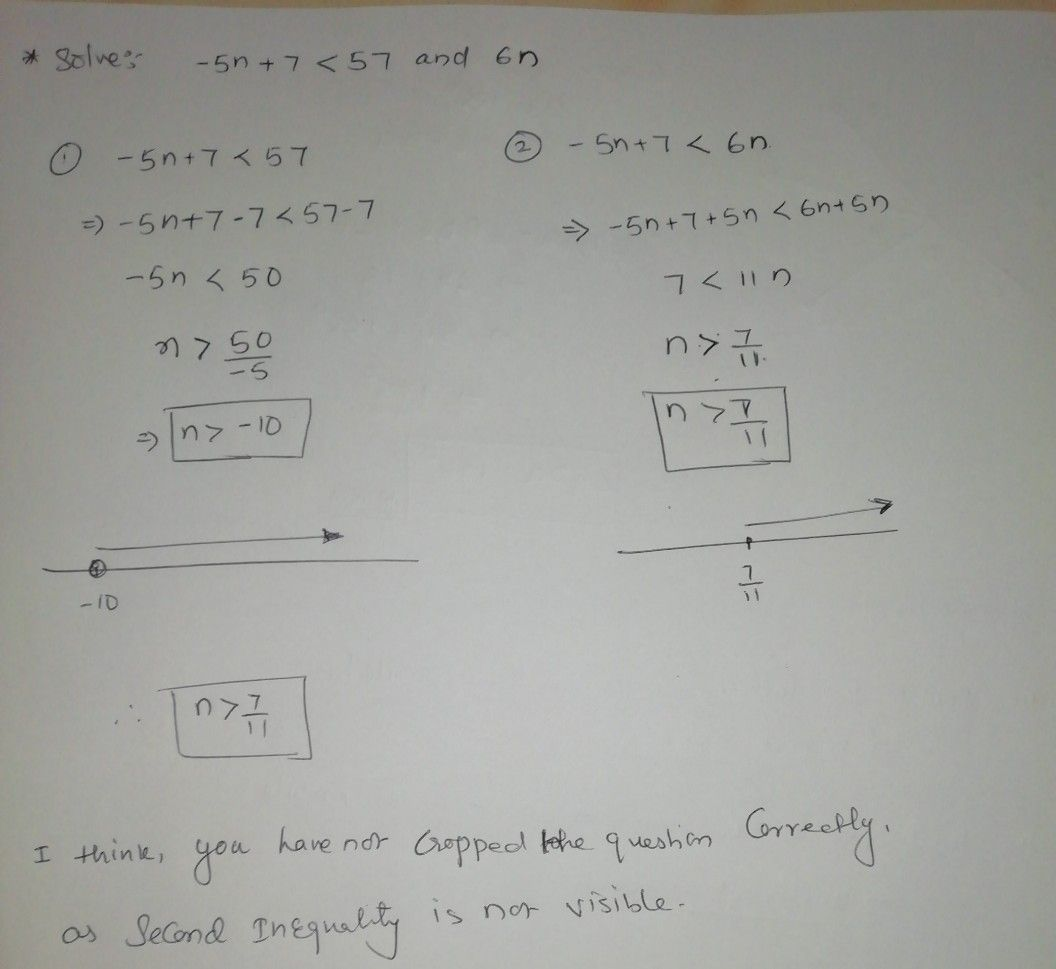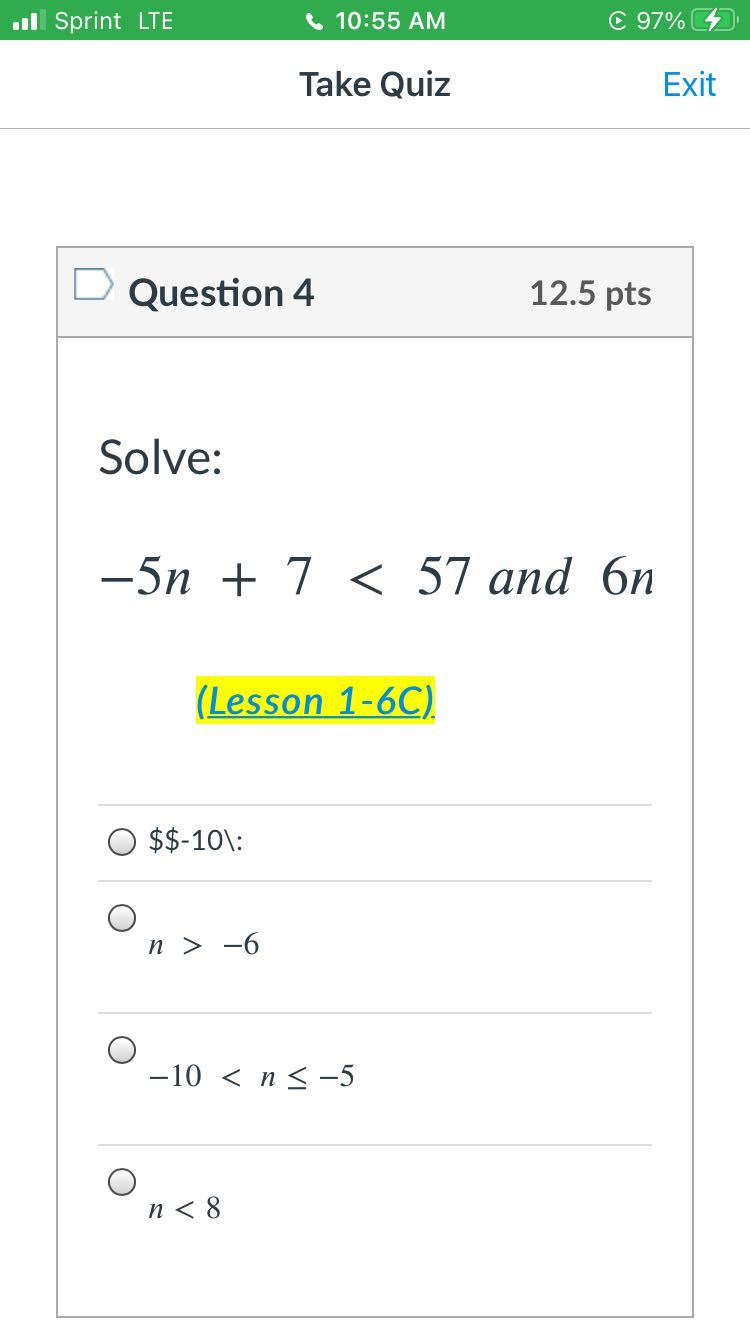Symbol
ProblemQuestion $4$ $12.5$ pts Solve: $-5n+7<57$ and $6n$ (Lesson $1-6C\right)$ $0s8-10:$ $0$ $n>-6$ $O$ $-10<n\leq -5$ $○$ $n<8$
Algebra
Question content
Please show the work on how you got the answers also this is “And”compound inequality
SolutionQanda teacher - anku1911I think you have not cropped the photo correctly, because second inequality is not visible.
I tried to solve, from whatever I can see.Student
That’s is the whole problemQanda teacher - anku1911
If you can, please crop the image again, and send it to me, so that I can help you solve it
Last part, where it says " and 6n".StudentThat^^^ is the problem I just made it bigger.Qanda teacher - anku1911
If that's the case, you can mark option C as answer. Coz no other option matches with first inequality
In cases of compound inequalities, we need two inequalities to solve. But here, second one on solving give n> 7/11
But you don't have any option which matches this condition